In :
import numpy as np, scipy.stats, pandas as pd, seaborn as sns, matplotlib.pyplot as plt
from matplotlib.cm import get_cmap
from matplotlib.patches import Patch
from sklearn.cluster import KMeans


Read in the Excel data. The Excel file contains two sheets, one of which is the transpose of the other. We want the first sheet, which Pandas reads by default.

In :
survey = pd.read_excel('survey.xlsx')

In :
survey.head(5)

Out:
Grade Class English is one of my favorite subjects. English is one of my most challenging subjects. I often don’t understand what’s going on in English class. I believe it’s important to learn English. I receive a lot of help from the other students. I find the other students distracting. If the teachers at our school were less strict, I would learn more. When I have a question, I tend to ask Max. ... More videos More games More partner/group activities More competitions More presentations More of something else Do you attend an English hagwon? Do you get one-on-one tutoring in English? Is there anything else you want Max to know? What temperature should we put the A/C at?
0 3 2 1.0 5.0 5.0 1.0 3.0 3.0 3.0 3.0 ... 5.0 5.0 4.0 2.0 2.0 NaN 0.0 0.0 NaN NaN
1 3 2 3.0 4.0 3.0 3.0 3.0 3.0 3.0 3.0 ... 4.0 4.0 3.0 3.0 3.0 NaN 0.0 0.0 NaN 18.0
2 3 2 3.0 4.0 3.0 5.0 3.0 4.0 3.0 4.0 ... 4.0 4.0 3.0 2.0 3.0 NaN 1.0 0.0 NaN NaN
3 3 2 4.0 3.0 2.0 5.0 3.0 4.0 1.0 3.0 ... 5.0 3.0 2.0 1.0 1.0 NaN 1.0 0.0 NaN 24.0
4 3 2 NaN NaN NaN NaN NaN NaN NaN NaN ... NaN NaN NaN NaN NaN NaN 0.0 0.0 NaN 20.0

5 rows × 21 columns

First we make a basic count plot showing how many responses there were to each question (154 surveys received total).

In :
pd.DataFrame(survey.count())

Out:
0
Class 154
English is one of my favorite subjects. 140
English is one of my most challenging subjects. 140
I often don’t understand what’s going on in English class. 139
I believe it’s important to learn English. 139
I receive a lot of help from the other students. 139
I find the other students distracting. 137
If the teachers at our school were less strict, I would learn more. 139
When I have a question, I tend to ask Max. 139
In general, Max is too lenient. 139
More videos 139
More games 136
More partner/group activities 135
More competitions 135
More presentations 133
More of something else 16
Do you attend an English hagwon? 146
Do you get one-on-one tutoring in English? 146
Is there anything else you want Max to know? 31
What temperature should we put the A/C at? 132

Now we compute t-scores using SciPy for all the agree/disagree questions. The null hypothesis is that the average is 3. We also compute p-values and flag for significance at the 0.05 level.

In :
results = pd.DataFrame(scipy.stats.ttest_1samp((survey.iloc[:,2:16]),3, nan_policy='omit')).T
results.index = survey.columns[2:16]
results.columns = ['T-score','p-value']
results['sig'] = results['p-value'] < 0.05
results

Out:
T-score p-value sig
English is one of my favorite subjects. -2.317269 2.194986e-02 True
English is one of my most challenging subjects. 3.698667 3.112224e-04 True
I often don’t understand what’s going on in English class. -0.260610 7.947818e-01 False
I believe it’s important to learn English. 9.045063 1.232238e-15 True
I receive a lot of help from the other students. 1.485556 1.396776e-01 False
I find the other students distracting. -10.515820 2.703568e-19 True
If the teachers at our school were less strict, I would learn more. -2.833684 5.292320e-03 True
When I have a question, I tend to ask Max. 0.699360 4.855040e-01 False
In general, Max is too lenient. 6.384556 2.436997e-09 True
More videos 16.546047 1.440986e-34 True
More games 13.993895 4.698432e-28 True
More partner/group activities -0.451455 6.523913e-01 False
More competitions -4.277926 3.563005e-05 True
More presentations -4.831435 3.701202e-06 True

Heat map of t-scores from above. This is better visualized, however, by the bar graph below.

In :
sns.set(context='talk',rc={'figure.figsize':(10,8)})
sns.heatmap(data=  results[['T-score']], # double brackets return it as a df instead of series
cmap=  'coolwarm',
annot= True,
vmin= -8.01,
vmax=  8.01 )

Out:
<matplotlib.axes._subplots.AxesSubplot at 0x113fae70>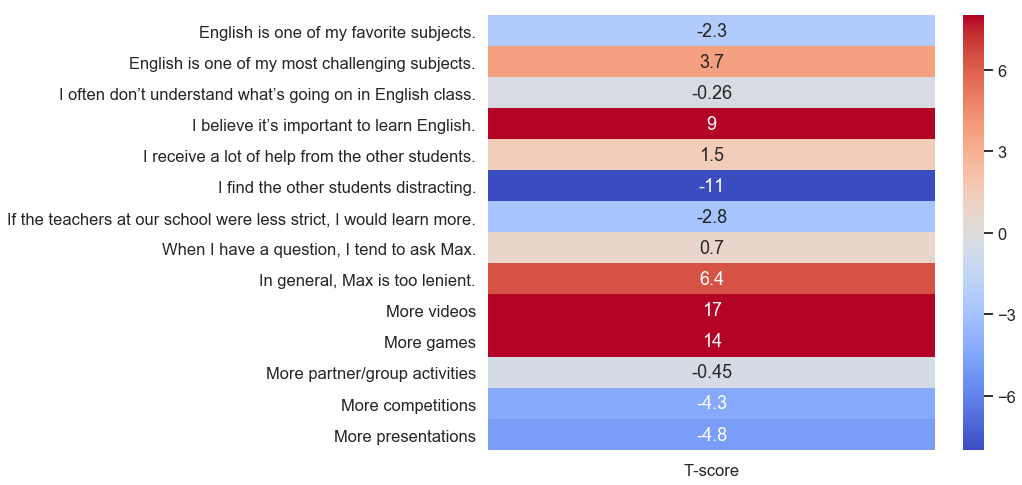I made two versions of this bar graph; the scale on the color map is wrong on the first one. See Stack Overflow link for discussion.

In :
# From a helpful guy on SE
# https://stackoverflow.com/questions/57601156/change-axis-along-which-pandas-applies-color-palette/57601461?noredirect=1#comment101680212_57601461
val_order = results['T-score'].rank(method='max').astype(int) # rank ordered values in column 't'
val_index = val_order - 1 # convert for use as a list index
colors = sns.color_palette('coolwarm', len(results.index)) # list of N colors spaced along coolwarm
palette = [colors[x] for x in val_index] # re-order colors with increasing value of 't'

sns.set(context='talk',rc={'figure.figsize':(10,8)})
sns.barplot(data=  results,
y=     results.index,
x=     'T-score',
palette= palette)

Out:
<matplotlib.axes._subplots.AxesSubplot at 0x117d1090>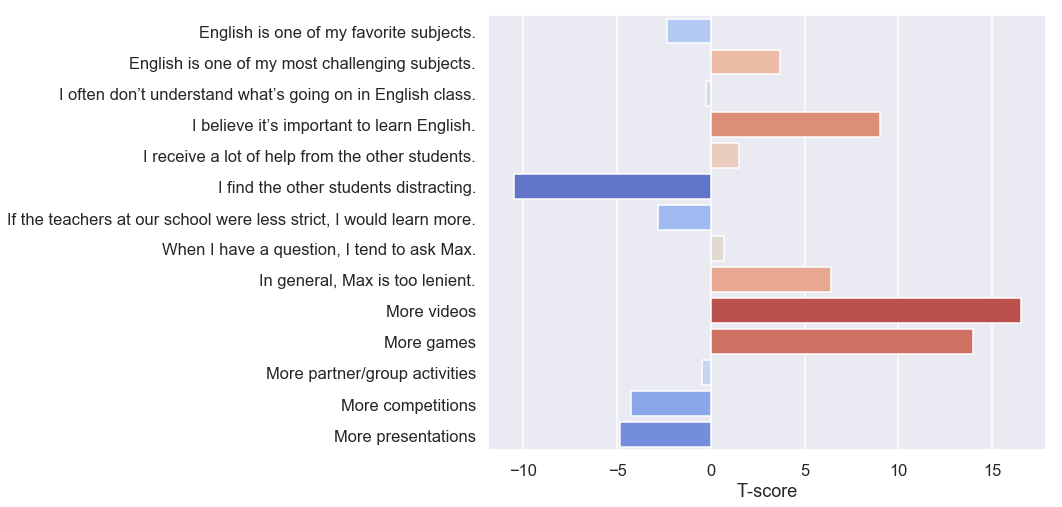In :
# better version, also from SE
sns.set(context='talk',rc={'figure.figsize':(10,8)})

absmax = np.abs(results['T-score'].values).max()
norm = plt.Normalize(-absmax, absmax)
cmap = plt.get_cmap("coolwarm")
colors = cmap(norm(results['T-score'].values))
plt.barh("index", "T-score", data=results.reset_index(), color=colors)

plt.gca().invert_yaxis()
plt.show()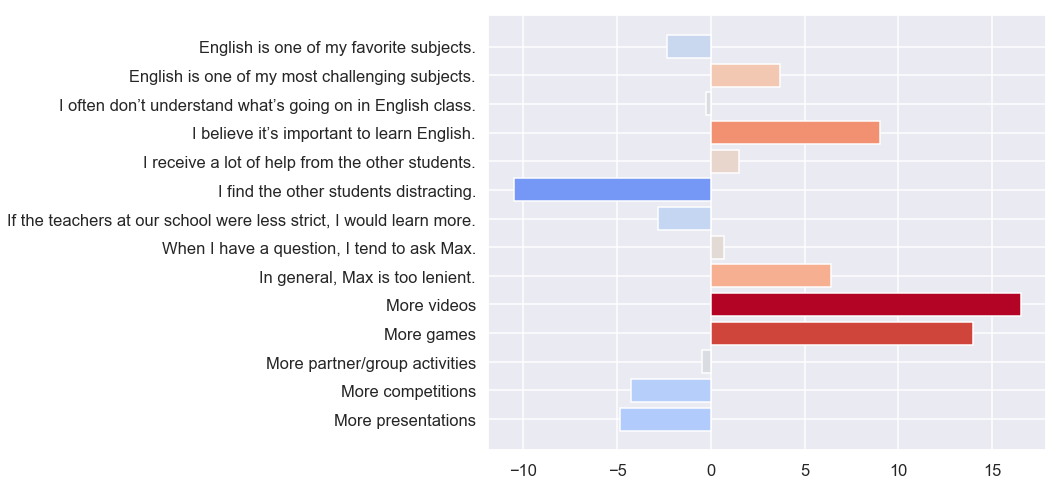As the above obscures differences between the classes and grades, we make a heatmap with the t-scores used as an aggregating function on a pivot table broken up by class section.

In :
classbreakdown = survey.iloc[:,:16].pivot_table(index=['Grade','Class'],
aggfunc=(lambda x: scipy.stats.ttest_1samp(x,3, nan_policy='omit')))
# Aggfunc above is computing t-scores centered around 3
classbreakdown = np.round(classbreakdown,decimals=1)
classbreakdown = classbreakdown[survey.columns[2:16]].T
# Restores original column order

In :
sns.set(context='talk',rc={'figure.figsize':(7,10)})
sns.heatmap(classbreakdown,cmap="coolwarm",cbar=False,vmin=-4,vmax=4,annot=True)
# sns.heatmap(classbreakdown.iloc[2:16],annot=True,vmin=1,vmax=5,cmap="coolwarm")
# Will just give normal averages

Out:
<matplotlib.axes._subplots.AxesSubplot at 0x1186b070>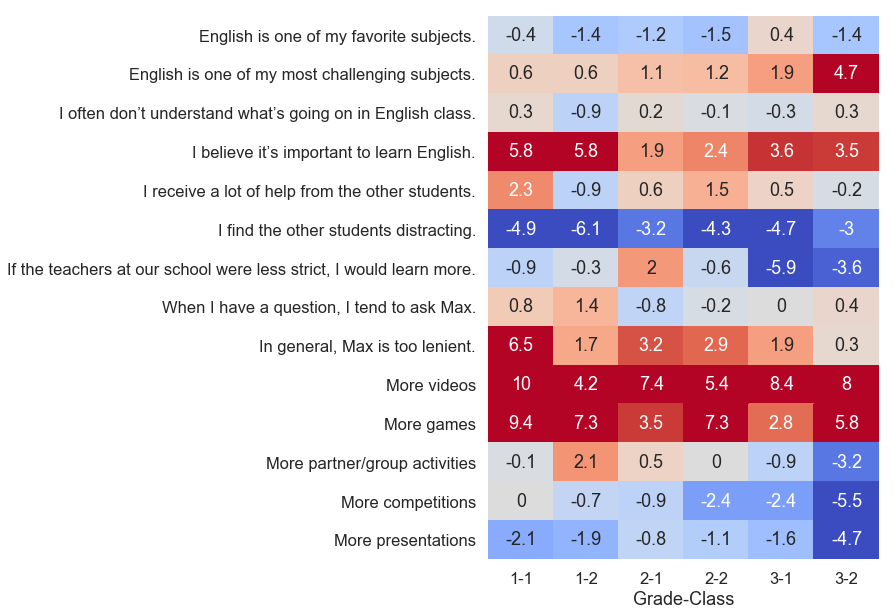Now we will compute r between all the quantitative questions. A Seaborn heatmap makes it easy to spot the action. Note especially the perceived importance column. Also negative correlation between hagwon and challenge, difficulty understanding. Lenience questions had little to do with perceived challenge or anything.

In :
sns.set(rc={'figure.figsize':(10,10)})
heatmap = sns.heatmap(np.round(survey.corr(),decimals=2),
cmap="coolwarm",
cbar=False,
vmin=-1,
vmax= 1,
annot=True)

# Code from SE that rotates the labels. I couldn't get it to work;
# labels keep clumping together on x axis.
# loc, labels = plt.xticks()
# heatmap.set_xticklabels(labels, rotation=45)
# heatmap.set_yticklabels(labels[::-1], rotation=45) # reversed order for y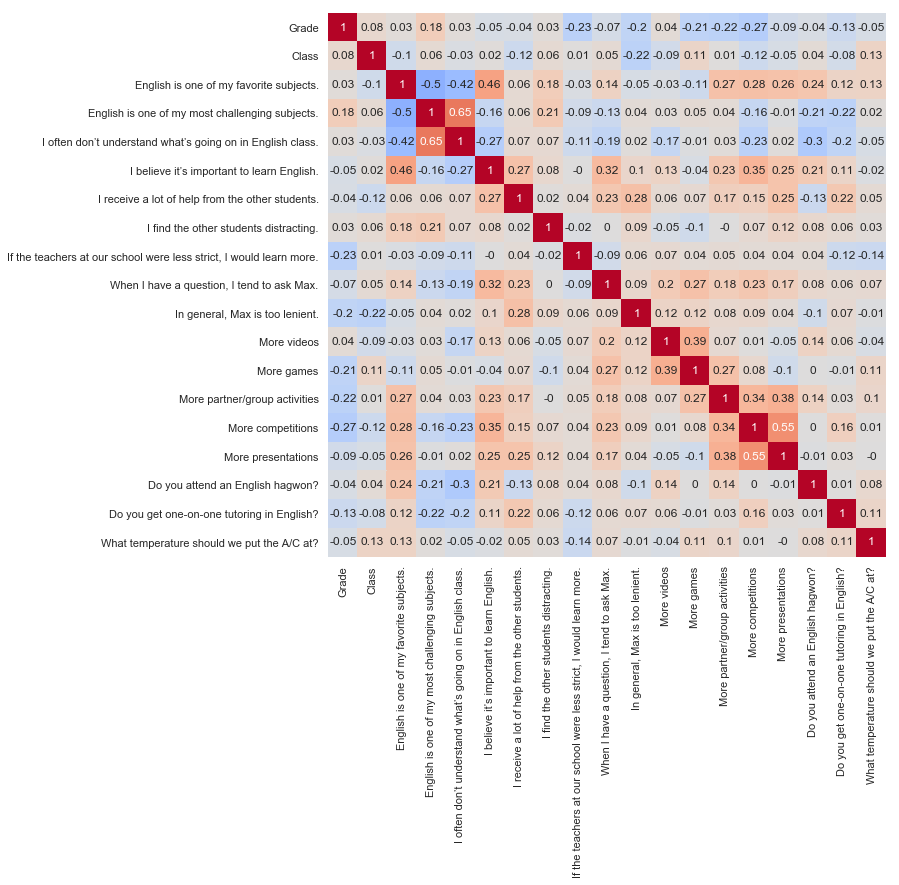This just illustrates that with so many dimensions, even random data will have some r values in the 0.2 range.

In :
# Random data
randcb = pd.DataFrame(np.random.rand(150,19)*4 + 1)

# Same plotting code
sns.set(rc={'figure.figsize':(10,10)})
heatmap = sns.heatmap(np.round(randcb.corr(),decimals=2),
cmap="coolwarm",
cbar=False,
vmin=-1,
vmax= 1,
annot=True)